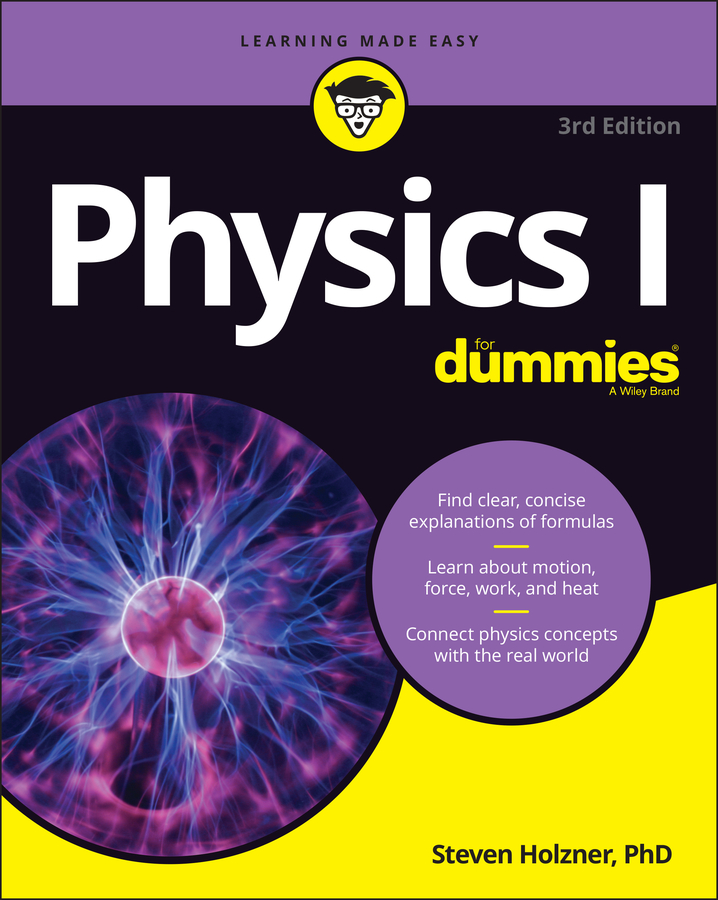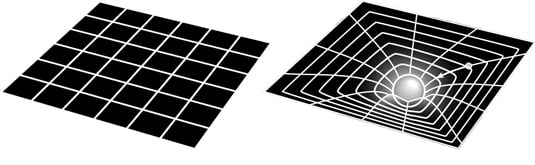##### Physics I For DummiesGeneral relativity was Einstein’s theory of gravity, published in 1915, which extended special relativity to take into account non-inertial frames of reference — areas that are accelerating with respect to each other. General relativity takes the form of field equations, describing the curvature of space-time and the distribution of matter throughout space-time. The effects of matter and space-time on each other are what we perceive as gravity.

The theory of the space-time continuum already existed, but under general relativity Einstein was able to describe gravity as the bending of space-time geometry. Einstein defined a set of field equations, which represented the way that gravity behaved in response to matter in space-time. These field equations could be used to represent the geometry of space-time that was at the heart of the theory of general relativity.

As Einstein developed his general theory of relativity, he had to refine the accepted notion of the space-time continuum into a more precise mathematical framework. He also introduced another principle, the principle of covariance. This principle states that the laws of physics must take the same form in all coordinate systems.

In other words, all space-time coordinates are treated the same by the laws of physics — in the form of Einstein’s field equations. This is similar to the relativity principle, which states that the laws of physics are the same for all observers moving at constant speeds. In fact, after general relativity was developed, it was clear that the principles of special relativity were a special case.

Einstein’s basic principle was that no matter where you are — Toledo, Mount Everest, Jupiter, or the Andromeda galaxy — the same laws apply. This time, though, the laws were the field equations, and your motion could very definitely impact what solutions came out of the field equations.

Applying the principle of covariance meant that the space-time coordinates in a gravitational field had to work exactly the same way as the space-time coordinates on a spaceship that was accelerating. If you’re accelerating through empty space (where the space-time field is flat, as in the left picture of this figure), the geometry of space-time would appear to curve. This meant that if there’s an object with mass generating a gravitational field, it had to curve the space-time field as well (as shown in the right picture of the figure).Without matter, space-time is flat (left), but it curves when matter is present (right).
In other words, Einstein had succeeded in explaining the Newtonian mystery of where gravity came from! Gravity resulted from massive objects bending space-time geometry itself.

Because space-time curved, the objects moving through space would follow the “straightest” path along the curve, which explains the motion of the planets. They follow a curved path around the sun because the sun bends space-time around it.

Again, you can think of this by analogy. If you’re flying by plane on Earth, you follow a path that curves around the Earth. In fact, if you take a flat map and draw a straight line between the start and end points of a trip, that would not be the shortest path to follow. The shortest path is actually the one formed by a “great circle” that you’d get if you cut the Earth directly in half, with both points along the outside of the cut. Traveling from New York City to northern Australia involves flying up along southern Canada and Alaska — nowhere close to a straight line on the flat maps we’re used to. Similarly, the planets in the solar system follow the shortest paths — those that require the least amount of energy — and that results in the motion we observe.

In 1911, Einstein had done enough work on general relativity to predict how much the light should curve in this situation, which should be visible to astronomers during an eclipse.

When he published his complete theory of general relativity in 1915, Einstein had corrected a couple of errors and in 1919, an expedition set out to observe the deflection of light by the sun during an eclipse, in to the west African island of Principe. The expedition leader was British astronomer Arthur Eddington, a strong supporter of Einstein.

Eddington returned to England with the pictures he needed, and his calculations showed that the deflection of light precisely matched Einstein’s predictions. General relativity had made a prediction that matched observation.

Albert Einstein had successfully created a theory that explained the gravitational forces of the universe and had done so by applying a handful of basic principles. To the degree possible, the work had been confirmed, and most of the physics world agreed with it. Almost overnight, Einstein’s name became world famous. In 1921, Einstein traveled through the United States to a media circus that probably wasn’t matched until the Beatlemania of the 1960s.